# Hooper's paradox facts for kids

Kids Encyclopedia Facts

Hooper's paradox is a falsidical paradox based on a optical illusion. A geometric shape with an area of 32 units is dissected into four parts, which afterwards get assembled into a rectangle with an area of only 30 units.

## Explanation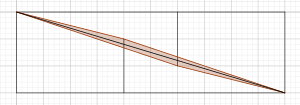Explanation of Hooper's paradox. The brown parallelogram is the overlapping area of the two triangles.

Upon close inspection one can notice that the triangles of the dissected shape are not identical to the triangles in the rectangle. The length of the shorter side at the right angle measures 2 units in the original shape but only 1.8 units in the rectangle. This means, the real triangles of the original shape overlap in the rectangle. The overlapping area is a parallelogram, the diagonals and sides of which can be computed via the Pythagorean theorem.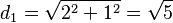$d_1=\sqrt{2^2+1^2}=\sqrt{5}$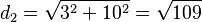$d_2=\sqrt{3^2+10^2}=\sqrt{109}$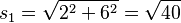$s_1=\sqrt{2^2+6^2}=\sqrt{40}$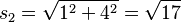$s_2=\sqrt{1^2+4^2}=\sqrt{17}$

The area of this parallelogram can determined using Heron's formula for triangles. This yields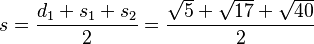$s=\frac{d_1+s_1+s_2}{2}=\frac{\sqrt{5}+\sqrt{17}+\sqrt{40}}{2}$

for the halved circumference of the triangle (half of the parallelogram) and with that for the area of the parallelogram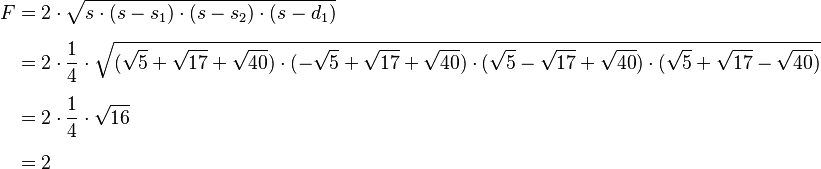\begin{align} F&=2\cdot \sqrt{s\cdot (s-s_1) \cdot (s-s_2) \cdot (s-d_1)} \\[5pt] &=2\cdot\frac{1}{4}\cdot\sqrt{(\sqrt{5}+\sqrt{17}+\sqrt{40})\cdot(-\sqrt{5}+\sqrt{17}+\sqrt{40})\cdot(\sqrt{5}-\sqrt{17}+\sqrt{40})\cdot(\sqrt{5}+\sqrt{17}-\sqrt{40})} \\[5pt] &=2\cdot\frac{1}{4}\cdot\sqrt{16} \\[5pt] &=2 \end{align}.

So the overlapping area of the two triangles accounts exactly for the vanished area of 2 units.Hooper's paradox Facts for Kids. Kiddle Encyclopedia.